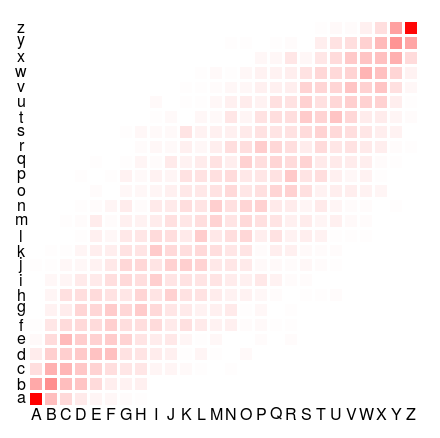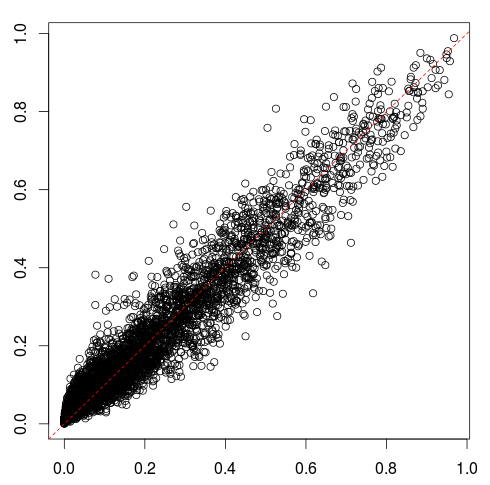Want to share your content on R-bloggers? click here if you have a blog, or here if you don't.

In econometric modeling, I usually have a problem with correlated features. A few weeks ago, I was discussing feature selection when features are correlated. This week, I was wondering about reverse engineering when features might be correlated (not to say very correlated). The way I see reverse engineering is the following1. someone has some dataset, and based on that dataset, a model was fitted. But we cannot see how it works….
2. we can generate “fake data”, feed the model with those data, and get predictions
3. based on those predictions, we wish we can fit a model that should be close to the the ‘true’ model used
4. one way to measure how good our model is is to compare predictions on the initial data with our model with the original dataset (or the initial ‘true’ values if we use generated datasets).

My concern was about those “fake data”, when features are correlated. My first concern was about the fact that if we generate randomly those fake data (without taking into accound correlations) there might be a huge was of time, since we will ask the model to fit a model on data that might not observed. For instance, if we have two factors,  and , that we assume to be extremely correlated, in the sense that only pairs  and  can be observed, we waste our time by generating the four possible pairs. My second concern was that those useless data might actually mislead our model. If half of the fake data cannot be obtained, but we still get a prediction, those might mislead our model, while we should focus on more valid data.

In order to test those intuitions, consider some simple (simulated dataset). We have two categorial variables (each of them has 26 levels), and a third one that is continuous. All those variables are correlated, in the sense that they were obtained from a latent joint Gaussian vector.

```> library(mnormt)
> n=5000
> V=matrix(c(1,.9,.7,.9,1,.8,.7,.8,1),3,3)
> #V=diag(c(1,1,1))
> Z=rmnorm(n,varcov=V)
> q=quantile(Z[,1],(0:26)/26)
> q=-5
> X1=cut(Z[,1],q,labels=LETTERS[1:26])
> q=quantile(Z[,2],(0:26)/26)
> q=-5
> X2=cut(Z[,2],q,labels=letters[1:26])
> Z3=Z[,3]
> Z1=Z[,1]
> Z2=Z[,2]
> X3=pnorm(Z3)
> mu=500+(Z1-Z2+Z3/2)*200
> Y=mu+rnorm(n)*10
> db=data.frame(Y,X1,X2,X3,mu)```

For instance, the heat map for the two categorical variables is the following,We recognize a Gaussian copula here, and there is some obvious “positive correlation” in the sense that we should have some  observations in our fake dataset, but a  would be a waste of time since it should be unlikely that we observe one.

Here, we did generate a standard linear model (a logistic regression will be considered afterwards).

`> reg=lm(Y~X1+X2+X3,data=db)`

To generate our fake dataset, we generate features independently.

```> ns=1000
> U1=sample(LETTERS[1:26],size=ns,replace=TRUE)
> U2=sample(letters[1:26],size=ns,replace=TRUE)
> U3=runif(ns,0,1)
> dfake=data.frame(X1=U1,X2=U2,X3=U3)
> Yf=predict(reg,newdata=dfake)```

and we get a prediction for those 1,000 fake data. Again, there is as much chance to get   than to get . We store the prediction we get from the (unknown) model, and we run a linear regression

```> dfake\$Y=Yf
> reg_reverse=lm(Y~X1+X2+X3,data=dfake)```

Actually, we might try also a random forest, or some boosting algorithm. But assume that we are lucky, at least, we try to fit the model in the same family. But on a very different dataset.

Now, if we compare the predictions we would get with that implied model with the ‘true’ values, we are not that bad

```> Y_reverse=predict(reg_reverse,newdata=db)
> plot(Y_reverse,db\$mu)
> abline(a=0,b=1,lty=2,col="red")```Of course, there were errors, but that is usually quite common. We can use the mean squared error to check the amount of error of our reverse engineering process, with the square of the difference between the prediction we would have with our model on the original dataset, and the true means used to generate the data.

Now, the question is about the impact of correlation. What could be the impact of having correlated data on the model we fit, and on the predictions. Consider the following functions (here, the latent Gaussian variables are exchangeable, with identical correlation)

```> mse=function(r=0){
+ n=5000
+ V=matrix(c(1,r,r,r,1,r,r,r,1),3,3)
+ Z=rmnorm(n,varcov=V)
+ q=quantile(Z[,1],(0:26)/26)
+ q=-5
+ X1=cut(Z[,1],q,labels=LETTERS[1:26])
+ q=quantile(Z[,2],(0:26)/26)
+ q=-5
+ X2=cut(Z[,2],q,labels=letters[1:26])
+ Z3=Z[,3]
+ Z1=Z[,1]
+ Z2=Z[,2]
+ X3=pnorm(Z3)
+ mu=500+(Z1-Z2+Z3/2)*200
+ Y=mu+rnorm(n)*10
+ db=data.frame(Y,X1,X2,X3)
+ reg=lm(Y~X1+X2+X3,data=db)
+ ns=1000
+ U1=sample(LETTERS[1:26],size=ns,replace=TRUE)
+ U2=sample(letters[1:26],size=ns,replace=TRUE)
+ U3=runif(ns,0,1)
+ dfake=data.frame(X1=U1,X2=U2,X3=U3)
+ Yf=predict(reg,newdata=dfake,type="response")
+ dfake\$Y=Yf
+ reg2=lm(Y~X1+X2+X3,data=dfake)
+ Y2=predict(reg2,newdata=db)
+ list(mse=mean((Y2-db\$Y)^2,
+ na.rm=TRUE),na=mean(is.na(Y2)))
+ }```

If we study the evolution of the mean square error as a function of the correlation, we have the following graph

```> M=matrix(unlist(lapply(seq(0,.95,by=.05),
+ mse)),nrow=2)[1,]
> plot(seq(0,.95,by=.05),M,type="l")```i.e. the mean squared error is actually decreasing as a function of the correlation. Which is clearly not the intuition I had initially….

What if we consider, instead, a classification problem, i.e. the variable of interest is obtained from a logistic regression

```> p=exp(-2+Z1-Z2+Z3/2)/(1+exp(-2+Z1-Z2+Z3/2))
> Y=rbinom(n,p,size=1)
> db=data.frame(Y,X1,X2,X3,p)
> reg=glm(Y~X1+X2+X3,family=binomial,data=db)
> dfake=data.frame(X1=U1,X2=U2,X3=U3)
> Yf=predict(reg,newdata=dfake,type="response")
> dfake\$Y=Yf
> reg_reverse=lm(Y~X1+X2+X3,data=dfake)
> Y_reverse=predict(reg_reverse,newdata=db)
> plot(Y_reverse,db\$p)
> abline(a=0,b=1,lty=2,col="red")```

Here, once we have our prediction (which is a probability) we fit a linear model, because we cannot fit a logistic regression (our prediction is not a class, here). The same process gives us the following prediction for the predicted probability, versus the true probability, used to generate the initial dataset.But we cannot really use it because our model returns negatative probabilities. So why not use a Generalized Linear Model, with a Gaussian distribution, but a logistic link function, to ensure that our prediction will be a probability

```> reg_reverse=glm(Y~X1+X2+X3,data=dfake,
> Y_reverse=predict(reg_reverse,newdata=db,
type="response")
> plot(Y_reverse,db\$p)
> abline(a=0,b=1,lty=2,col="red")```Again, our model performs quite well. To check the impact of the correlation, use

```> mse=function(r=0){
+ n=5000
+ V=matrix(c(1,r,r,r,1,r,r,r,1),3,3)
+ Z=rmnorm(n,varcov=V)
+ q=quantile(Z[,1],(0:26)/26)
+ q=-5
+ X1=cut(Z[,1],q,labels=LETTERS[1:26])
+ q=quantile(Z[,2],(0:26)/26)
+ q=-5
+ X2=cut(Z[,2],q,labels=letters[1:26])
+ Z3=Z[,3]
+ Z1=Z[,1]
+ Z2=Z[,2]
+ X3=pnorm(Z3)
+ mu=500+(Z1-Z2+Z3/2)*200
+ p=exp(-2+Z1-Z2+Z3/2)/(1+exp(-2+Z1-Z2+Z3/2))
+ Y=rbinom(n,p,size=1)
+ db=data.frame(Y,X1,X2,X3)
+ reg=glm(Y~X1+X2+X3,family=binomial,data=db)
+ ns=1000
+ U1=sample(LETTERS[1:26],size=ns,replace=TRUE)
+ U2=sample(letters[1:26],size=ns,replace=TRUE)
+ U3=runif(ns,0,1)
+ dfake=data.frame(X1=U1,X2=U2,X3=U3)
+ Yf=predict(reg,newdata=dfake,type="response")
+ dfake\$Y=Yf
+ reg2=glm(Y~X1+X2+X3,data=dfake,
+ Y2=predict(reg2,newdata=db,type="response")
+ list(mse=mean((Y2-db\$Y)^2,
+ na.rm=TRUE),na=mean(is.na(Y2)))
+ }```

and return – here again – the mean squared error.

```> M=matrix(unlist(lapply(seq(0,.95,by=.05),mse)),
+ nrow=2)[1,]
> plot(seq(0,.95,by=.05),M,type="l")```

and again, the error is decreasing with the correlation.It looks like having correlated data is actually a good thing. The intuition might be that if the three variables are very correlated, then then model behaves as if there was only onvariable, so there should be less variability. But still. Here , with high correlation, we generate a lot a non-relevant fake data… which might be misleading our implied model…. So here, I have to admit that I find that output quite puzzling.

What if we use a wrong model family, e.g. some random forest model

```> mse_rf=function(r=0){
+   n=5000
+   V=matrix(c(1,r,r,r,1,r,r,r,1),3,3)
+   Z=rmnorm(n,varcov=V)
+   q=quantile(Z[,1],(0:26)/26)
+   q=-5
+   X1=cut(Z[,1],q,labels=LETTERS[1:26])
+   q=quantile(Z[,2],(0:26)/26)
+   q=-5
+   X2=cut(Z[,2],q,labels=letters[1:26])
+   Z3=Z[,3]
+   Z1=Z[,1]
+   Z2=Z[,2]
+   X3=pnorm(Z3)
+   mu=500+(Z1-Z2+Z3/2)*200
+   p=exp(-2+Z1-Z2+Z3/2)/(1+exp(-2+Z1-Z2+Z3/2))
+   Y=rbinom(n,p,size=1)
+   db=data.frame(Y,X1,X2,X3)
+   reg=glm(Y~X1+X2+X3,family=binomial,data=db)
+   ns=1000
+   U1=sample(LETTERS[1:26],size=ns,replace=TRUE)
+   U2=sample(letters[1:26],size=ns,replace=TRUE)
+   U3=runif(ns,0,1)
+   dfake=data.frame(X1=U1,X2=U2,X3=U3)
+   Yf=predict(reg,newdata=dfake,type="response")
+   dfake\$Y=Yf
+   reg2=randomForest(Y~X1+X2+X3,data=dfake)
+   Y2=predict(reg2,newdata=db,type="response")
+   list(mse=mean((Y2-db\$Y)^2,na.rm=TRUE),
+   na=mean(is.na(Yencore)))
+ }```

Here, the graph of the mean squared error, as a function of the correlation is also decreasing….To conclude that long post, I think I now face two alternatives. Either my intuition is not valid (which is rather possible actually). Or the measure I use is not valid. To be more specific, I measure here the mean squared error, with various correlations. But in that case (for instance the initial linear model), the sum of the latent variable has a changing range when correlation is changing; So the range of  is also changing with the correlation. So using that metric is maybe not very clever…. And next time, I will try to generate fake data that actually incorporate that correlation. If my understanding is correct, I should get a much lower error, and a much better model.